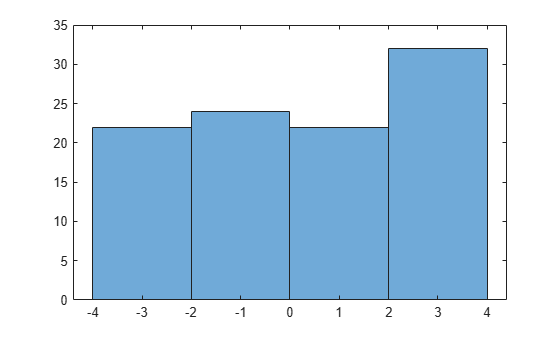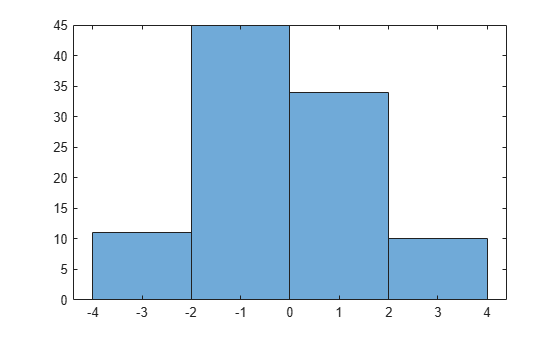Documentation

This is machine translation

Mouseover text to see original. Click the button below to return to the English version of the page.

randsrc

Generate random matrix using prescribed alphabet

Syntax

``out = randsrc``
``out = randsrc(m)``
``out = randsrc(m,n)``
``out = randsrc(m,n,alphabet)``
``out = randsrc(m,n,[alphabet; prob])``
``out = randsrc(m,n,___,seed)``
``out = randsrc(m,n,___,streamhandle)``

Description

````out = randsrc` generates a random scalar that is either `-1` or `1`, with equal probability.```
````out = randsrc(m)` generates an `m`-by-`m` random bipolar matrix. Each entry independently takes the value `-1` or `1` with equal probability.```
````out = randsrc(m,n)` generates an `m`-by-`n` random bipolar matrix. Each entry independently takes the value `-1` or `1` with equal probability.```
````out = randsrc(m,n,alphabet)` generates an `m`-by-`n` matrix, with each entry independently chosen from the entries in the row vector `alphabet`. Each entry in `alphabet` occurs in `out` with equal probability. Duplicate values in `alphabet` are ignored.```
````out = randsrc(m,n,[alphabet; prob])` generates an `m`-by-`n` matrix, with each entry independently chosen from the entries in the row vector `alphabet`. Duplicate values in `alphabet` are ignored. The row vector `prob` lists corresponding probabilities, so that the symbol `alphabet(k)` occurs with probability `prob(k)`, where `k` is any integer between one and the number of columns of `alphabet`. The elements of `prob` must add up to 1.```
````out = randsrc(m,n,___,seed)` accepts input combinations from prior syntaxes and a seed value, for initializing the uniform random number generator, `rand`. ```
``` `out = randsrc(m,n,___,streamhandle)` accepts input combinations from prior syntaxes and a random stream handle to generate uniform random noise samples by using `rand`. Providing a random stream handle or using the `reset` function on the default random stream object enables you to generate repeatable noise samples. If you want to generate repeatable noise samples, then either reset the random stream input before calling `randsrc` or use the same seed input. For more information, see `RandStream`.```

Examples

collapse all

Generate a 10-by-10 matrix from the set of {-3,-1,1,3}.

`out = randsrc(10,10,[-3 -1 1 3])`
```out = 10×10 3 -3 1 1 -1 -1 3 3 -1 -3 3 3 -3 -3 -1 1 -1 -1 3 -3 -3 3 3 -1 3 1 1 3 1 1 3 -1 3 -3 3 -3 1 -3 1 3 1 3 1 -3 -3 -3 3 3 3 3 -3 -3 3 3 -1 -1 3 -1 -1 -3 -1 -1 1 1 -1 3 1 -3 3 1 1 3 -1 -1 1 -1 -3 -1 3 -1 3 3 1 3 1 1 -3 1 -1 -3 3 3 -3 -3 3 -3 -1 -1 1 -1 ```

Plot the histogram. Each of the four possible element values occur with equal probability. Your values might differ.

`histogram(out,[-4 -2 0 2 4])`Generate a matrix in which the likelihood of a -1 or 1 is four times higher than the likelihood of a -3 or 3.

`out = randsrc(10,10,[-3 -1 1 3; 0.1 0.4 0.4 0.1])`
```out = 10×10 -1 -1 -1 -1 1 -1 1 -1 1 3 1 -3 3 3 1 -3 -1 -1 -1 1 -1 -1 -3 -1 -1 3 -1 1 1 -1 1 3 1 -1 1 3 -1 -3 -1 -1 -1 -1 1 -1 -1 -1 -3 -3 1 -1 1 1 1 -1 -3 -1 -1 -1 -1 -1 -1 1 -3 1 -1 -1 3 1 -1 1 1 3 -1 1 -1 3 3 1 1 1 1 -3 -1 1 -1 -1 1 1 1 1 1 -1 1 -1 -1 -1 -3 -1 -3 1 ```

Plot the histogram. Values of -1 and 1 are more likely.

`histogram(out,[-4 -2 0 2 4])`